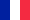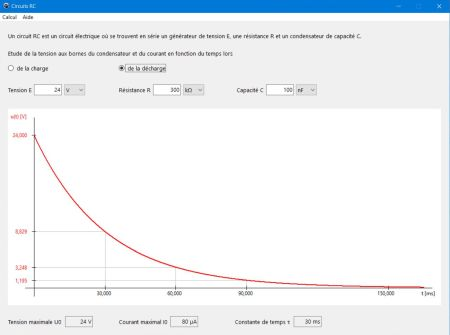# Computing: Free Pascal Programming

## Capacitor charge and discharge.Description: Electronics related application, studying the voltage and the current as a function of time during charge/discharge of a capacitor. To do the calculations:

• choose charge resp. discharge, by checking the corresponding radiobutton;
• fill in the values, defining the circuit: voltage E, resistance R and capacity C (in the case of charge, E = voltage of the generator; in the case of discharge, E = capacitor voltage when discharge begins = maximum voltage when charging with same values for R and C);
• choose u(t), i(t) or u(t) and i(t) (to draw both curves on the same graph) in the Calcul menu to start the calculation.
The program calculates Umax, Imax and the time constant τ, choosing the units in a way that their values may be represented "as small numbers with just 3 fractional digits". The u(t) or i(t) curve is drawn from t = 0 to t = 5τ, where the capacitor may be considered completely charged/discharged.
Help with the physics of capacitor circuits as well as with the usage of the application (in French as the application itself) is included.

To do: Display of both curves on the same graph in the case of a discharge.

Free Pascal features: Using bitmap canvas to draw mathematics function graphs. Displaying help using a TMemo object on a second form; filling a TMemo object from a text file.

Screenshots: